Refer to our Texas Go Math Grade 6 Answer Key Pdf to score good marks in the exams. Test yourself by practicing the problems from Texas Go Math Grade 6 Module 14 Quiz Answer Key.

14.1 Graphing on the Coordinate Plane

Graph each point on the coordinate plane.

Question 1.
A(-2, 4)
Point A is 2 units left of the origin, and 4 units up. It has x-coordinate -2 and y-coordinate 4 written (-2, 4). It is located in Quadrant II.
A = (-2, 4) Final solution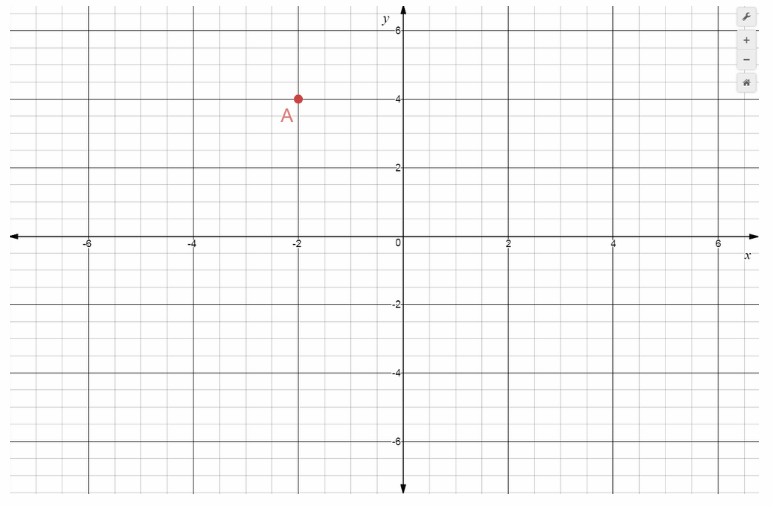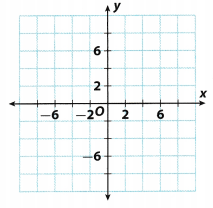Question 2.
B(3, 5)
Point B is 3 units right of the origin, and 5 units up. It has x-coordinate 3 and y-coordinate 5, written (3, 5). It is located in Quadrant I.
B = (3, 5) Final solutionQuestion 3.
C(6, -4)
Point C is 6 units right of the origin, and 4 units down. It has x-coordinate 6 and y-coordinate -4 written (6, -4) It is located in Quadrant IV.
C = (6, -4) Final solution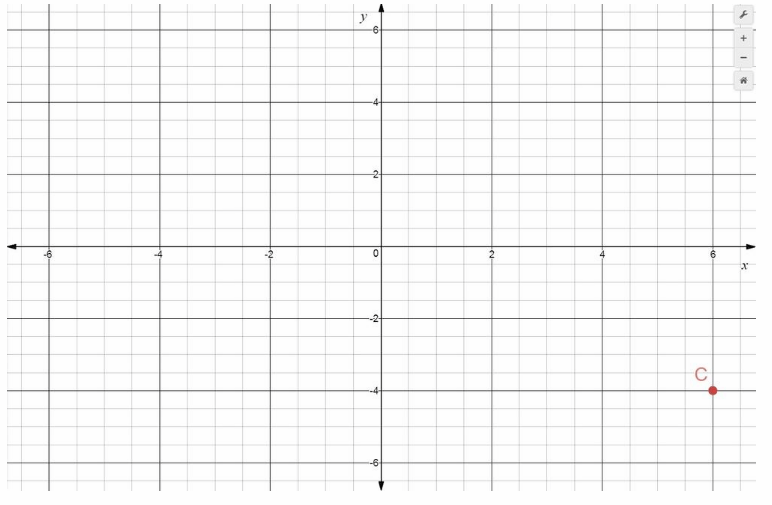Question 4.
D(-3, -5)
Point D is 3 units left of the origin, and 5 units down. It has x-coordinate -3 and y-coordinate -5, written (-3, -5) It is located in Quadrant III.
D(-3, -5) Final solutionQuestion 5.
E(7, 2)
Point E is 7 units right of the origin, and 2 units up It has x-coordinate 7 and y-coordinate 2, written (7, 2). It is located in Quadrant I.
E = (7, 2) Final solution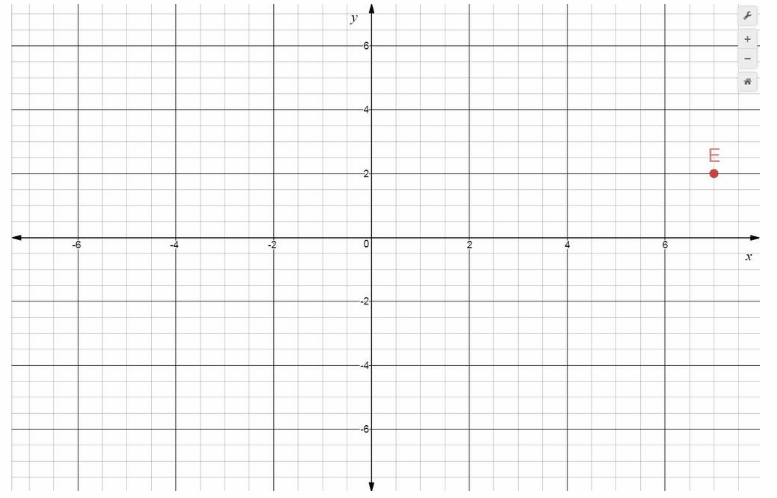Question 6.
F(-4, 6)
Point F is 4 units left of the origin, and 6 units up It has x-coordinate -4 and y-coordinate 6, written (-4, 6). It is located in Quadrant II.
F = (-4, 6) Fina solution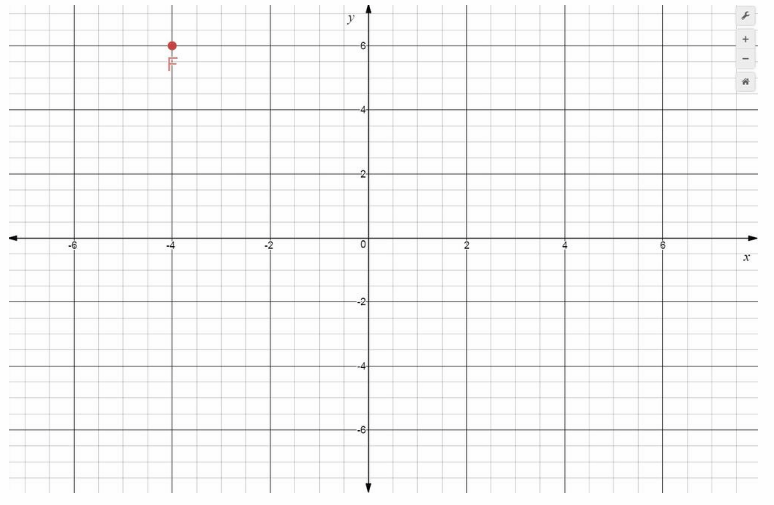14.2 Independent and Dependent Variables in Tables and Graphs

Question 7.
Jon buys packages of pens for $5 each. Identify the dependent variables in the situation. Answer: The independent variable in this situation is the number of packages bought. The dependent variable in this situation is the total cost of the packages. The equation relating the 2 is y = 5x 14.3 Writing Equations from Tables Write an equation that represents the data in the table. Question 8.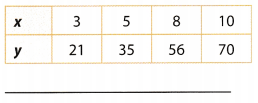Answer: Compare the x- and y-values to find a pattern Each y-value is 7 times the corresponding x-value Use the pattern to write an equation expressing y in terms of x y = 7x y = 7x Final solution y = 7x Question 9.Answer: Compare the x- and y-values to find a pattern. Each y-value is l2more than the corresponding x-value. Use the pattern to write an equation expressing y in terms of x. y = x + 12 y = x + 12 Final solution y = x + 12 14.4 Representing Algebraic Relationships in Tables and Graphs Graph each equation. Question 10. y = x + 3Answer: Make a table of values. Choose some values for x and use the equation to find the corresponding values for y. Plot the ordered pairs from the table. Draw a line through the plotted points to represent all of the ordered pair solutions of the equation.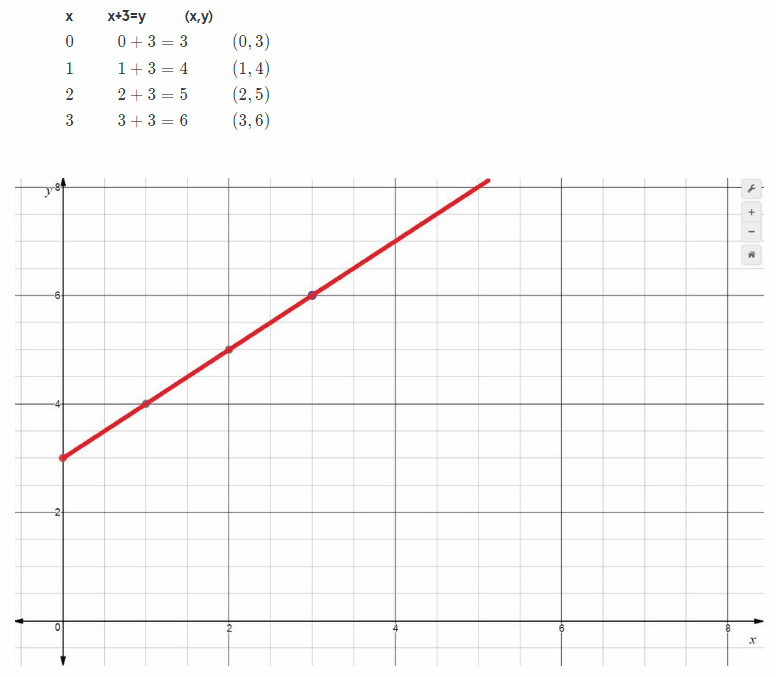Question 11. y = 5x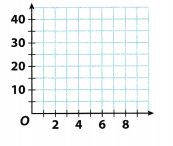Answer: Solution to this example is given below y = 5x Make a table of values. Choose some values for x and use the equation to find the corresponding values for y. Plot the ordered pairs from the table. Draw a line through the plotted points to represent all of the ordered pair solutions of the equation.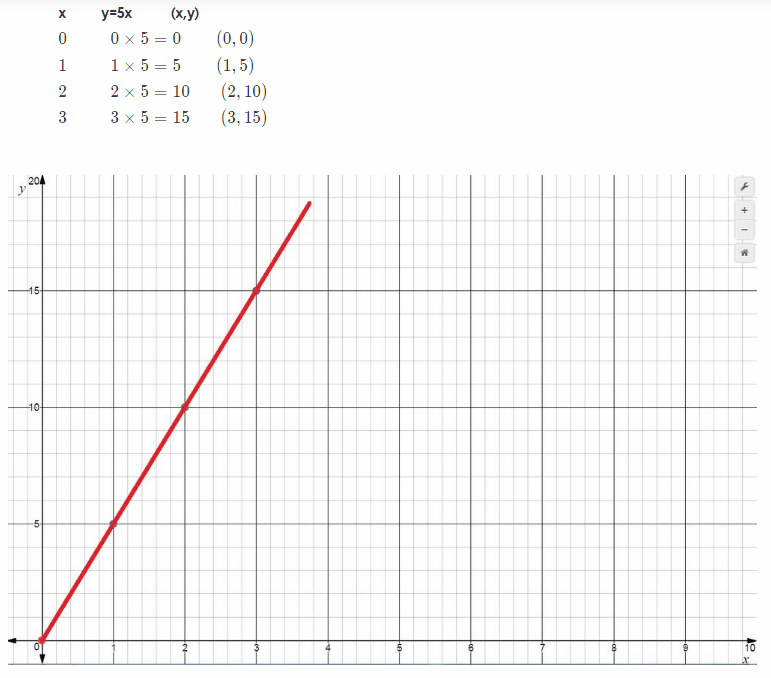Essential Question Question 12. How can you write an equation ¡n two variables to solve a problem? Answer: Any 2 quantity based situation can be converted to an algebraic equation by identifying the independent and the dependent quantity and examining the relationship between them Texas Go Math Grade 6 Module 14 Mixed Review Texas Test Prep Answer Key Selected Response Question 1. What are the coordinates of point G on the coordinate grid below?(A) (4, 3) (B) (4, -3) (C) (-4, 3) (D) (-4, -3) Answer: (B) (4, -3) Explanation: Point G is 4 units right on the origin, and 3 units down It has x-coordinate 4 and y-coordinate -3, written (4, -3). It is located in Quadrant IV. (G = 4, -3) B This option is correct answer Question 2. A point is located in quadrant II of a coordinate plane. Which of the following could be the coordinates of that point? (A) (-5, -7) (B) (5, 7) (C) (-5, 7) (D) (5, -7) Answer: (C) (-5, 7) Explanation: A) Point A is 5 units Left of the origin, and 7 units down It has x-coordinate -5 and y-coordinate -7, written (-5, -7). It is located in Quadrant III. Option A) is not correct answer B)Point B is 5 units right of the origin, and 7 units up. It has x-coordinate 5 and y-coordinate 7, written (5, 7). It is located in Quadrant I. Option B) is not correct answer C)Point C is 5 units Left of the origin, and 7 units up. It has x-coordinate -5 and y-coordinate 7, written (-5, 7). It is located in Quadrant II. Option C) is correct answer D)Point D is 5 units right of the origin, and 7 units down. It has x-coordinate 5 and y-coordinate -7, written (5, -7). It is located in Quadrant IV. Option D) is not correct answer C This option is correct answer Question 3. Man had 5 library books. He checked 1 additional book out every week without returning any books. Which equation describes the number of books he has, y, after x weeks? (A) y = 5x (B) y = 5 – x (C) y = 1 + 5x (D) y = 5 + x Answer: (D) y = 5 + x Explanation: He had 5 books which increased at the rate of 1 book per week so he had y = x + 5 books in x weeks Option D Question 4. Stewart is playing a video game. He earns the same number of points for each prize he captures. He earned 1,200 points for 6 prizes, 2,000 points for 10 prizes, and 2,600 points for 13 prizes. Which is the dependent variable in the situation? (A) the number of prizes captured (B) the number of points earned (C) the number of hours (D) the number of prizes available Answer: (B) the number of points earned Explanation: The number of points earned depends on the number of prizes captured, therefore the number of points earned is the dependent variable. Option B. Question 5. Dwayne graphed the equation y = 10 + x. Which point does the graph not pass through? (Â) (0, 10) (B) (3, 13) (C) (8, 2) (D) (5, 15) Answer: (C) (8, 2) Explanation: We notice that through the point (8, 2) the graph does not pass. Really if we substitute 8 for r in the equation, we get: y = 10 + 8 = 18 ≠ 2 So, the conclusion is that correct answer is C (C) (8, 2) Question 6. Amy gets paid by the hour. Her little sister helps. As shown below, Amy gives her sister part of her earnings. Which equation represents Amy’s pay when her sister’s pay is$13?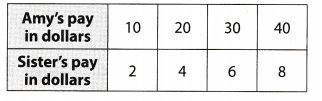(A) y = $$\frac{13}{5}$$
(B) 13 = $$\frac{x}{5}$$
(C) 5y = 13
(D) 13 = 5x
First, we need to find an equation which describes this situation: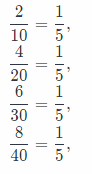so, we get the following equation
y = $$\frac{1}{5}$$x
Now, we will substitute 13 for y in previous equation and we wilL find the equation which represent Amy’s pay
13 = $$\frac{x}{5}$$x

Gridded Response

Question 7.
Betty earns $7.50 per hour at a part-time job. Let x be the number of hours and y be the amount she earns. Betty makes a graph to show how x and y are related. If she earns$60, how many hours did she work?